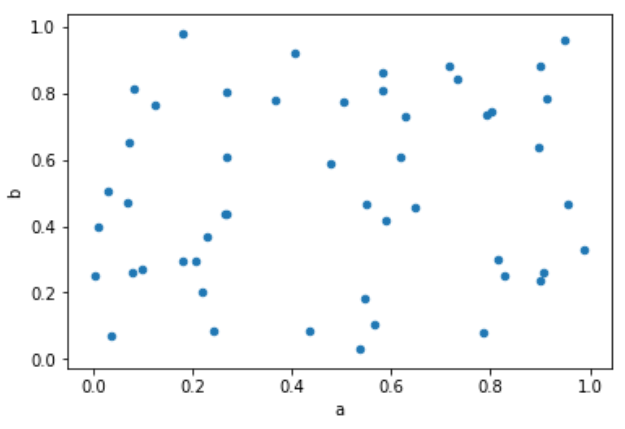# Python – Scatter Plots

Scatterplots show many points plotted in the Cartesian plane. Each point represents the values of two variables. One variable is chosen in the horizontal axis and another in the vertical axis.

## Drawing a Scatter Plot

Scatter plot can be created using the DataFrame.plot.scatter() methods.

```import pandas as pd
import numpy as np
df = pd.DataFrame(np.random.rand(50, 4), columns=['a', 'b', 'c', 'd'])
df.plot.scatter(x='a', y='b')
```

Its output is as follows −